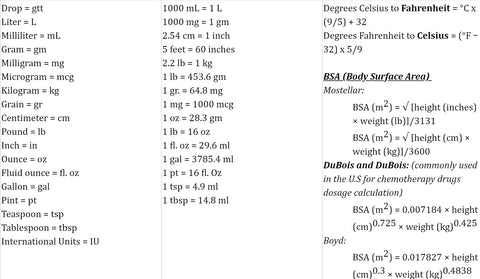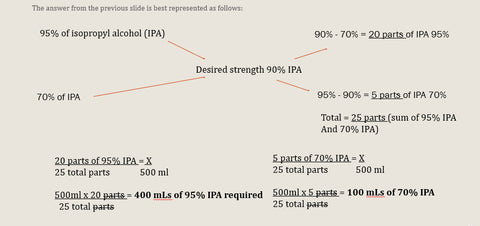# Sterile Compounding Certification Pharmacy Math Calculations

NEED TO KNOW STERILE COMPOUNDING MATH BASICSCONCENTRATION CALCULATION FORMULAS

Alligation MethodPERCENTAGE CONCENTRATION

For solids %w/w = gm/100 gm   w = weight

For liquids %v/v = ml/100 ml      v = volume

For a mix of solid and liquid %w/v = gm/100 ml

1% Hydrocortisone (HC) cream contains 1g of HC per 100g of cream.

A 1% solution = 1ml of active ingredient per 100ml of the solution.

A 3% w/v solution = 3g of solid per 100 ml of  the solution.

RATIO STRENGTH

Ratios…

1.Compare 2 numbers in relation to each other ¼ = 1:4

AND

2. When added together make a whole - 1:2:2 = total of 5 parts

Example

Two solutions are combined in a 2:1 ratio to make a final solution. How many mLs of each solution is needed to make 100 ml of the final solution?

In this case, you would need to solve this question using #2 above.

1. Total parts = 3 (2+1)

2. mLs needed for the numerator (top number) = 2/3 x 100 ml = 66.67 mLs

3. mLs needed for the denominator = 1/3 x 100 ml = 33.33 mLs

The total from 2 & 3 above should equal 100 mLs.

DILUTIONS

To change the strength of a preparation, adding a diluent to it is necessary which would result in the final preparation having a lower percent strength. The most common formula used for liquids and solids is:

V1 x S1 = V2 x S2 (V = Volume and S = Strength (may be entered as whole numbers).

Example: How many mLs of a 0.5% stock solution (SS) is required to make 1.5 L of a 0.05% solution using water as a solvent?

As in proportions, 3 variables must be known to solve for the fourth. Leave percent strength as shown.  So for this example….

V1 = ?    S1 = 0.5%    V2 = 1.5L = 1500ml    S2 = 0.05%

So...........

V1 x S1 = V2 x S2

V1 x 0.5% = 1500ml (1.5 L) x 0.05%

V1 = 1500ml x 0.05%

0.5%

V1 = 150 ml of stock solution (SS) required.

From above, you know the diluent or solvent is water so 1500 (total) – 150 (SS) = 1350 ml of water required.

For more math equations such as IV flow rates, dosing calculations and more, sign up for the standalone sterile compounding course or sign up for the IV certification program.